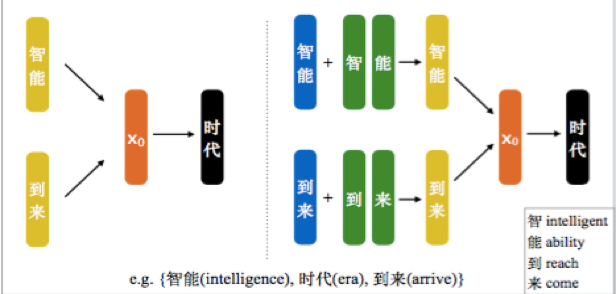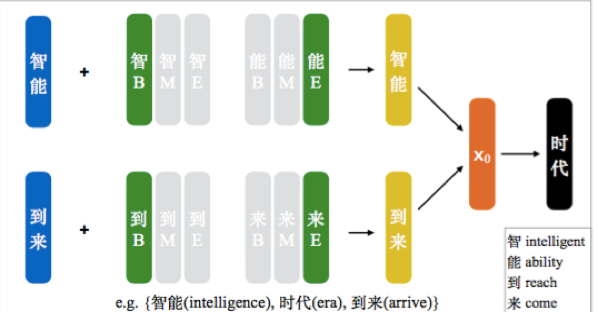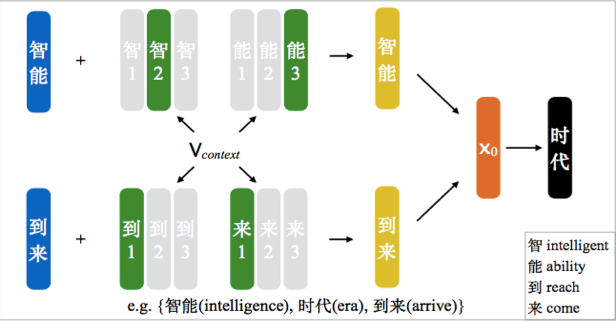# 字和词语联合训练的词向量模型

Nki=klogPr(xi|xik,....,xi+k)$\sum_{i=k}^{N-k}logPr(x_i|x_{i-k},....,x_{i+k})$
Pr(xi|xik,....,xi+k)=exp(xo.xi)xjDictionaryexp(xo.xj)$Pr(x_i|x_{i-k},....,x_{i+k})=\frac{exp(x_o.x_i)}{\sum_{x_j\in{Dictionary}}exp(x_o.x_j)}$
xo=12kj=ik,....i+kxj$x_o=\frac{1}{2k}\sum_{j=i-k,....i+k}x_j$xj=wj1NjNjk=1ck$x_j=w_j\bigoplus\frac{1}{N_j}\sum_{k=1}^{N_j}c_k$

$\bigoplus$对应的操作有拼接和相加两种方式，paper里说拼接方式虽然增加了模型的复杂度，但是对于效果的提升并不明显，因此后面的模型中直接就采用了相加的方式，公式如下所示：
xj=12(wj+1NjNjk=1ck)$x_j=\frac{1}{2}(w_j+\frac{1}{N_j}\sum_{k=1}^{N_j}c_k)$

1 Position-based Character Embedding2 Cluster_based Character Embeddingrmaxk=argmaxrkcos(vcontext,crkk)$r_k^{max}=arg max_{r_k}cos(v_{context},c_k^{r_k})$
vcontext=j+kt=jkxt=j+kt=jk12(wt+1Ntcuxtcmostu)$v_{context}=\sum_{t=j-k}^{j+k}x_t=\sum_{t=j-k}^{j+k}\frac{1}{2}(w_t+\frac{1}{N_t}\sum_{c_u\in{x_t}}c_u^{most})$
xj=12(wj+1NjNjk=1crmaxkk)$x_j=\frac{1}{2}(w_j+\frac{1}{N_j}\sum_{k=1}^{N_j}c_k^{r_k^{max}})$

3 Nonparametric Cluster-based Character Embeddings

if(cos(vcontext,vrkk)<γ$cos(v_{context},v_k^{r_k})<\gamma$)
rk=new vector$r_k=new\ vector$
else
rk=rmaxk$r_k=r_k^{max}$

1 本文提出的汉字和词语结合的方式就是简单的向量相加操作，其实也许应用矩阵变换等方式可以把二者更加合理地结合在一起；
2 在相加的时候，需要给以每一个汉字不同权重，这也和我之前说的一致，可以利用attention机制，只不过在2015年的时候还没有attention的概念。

ps:最后这篇paper一句话解决我的一个困惑，就是为什么大家再生成词向量的时候选择的方法从来都不考虑Bengio刚开始提出的那个比较复杂的模型：“The training process of most previous word embedding models exhibits high computational complexity, which makes them unable to work for large-scale text corpora efficiently.”